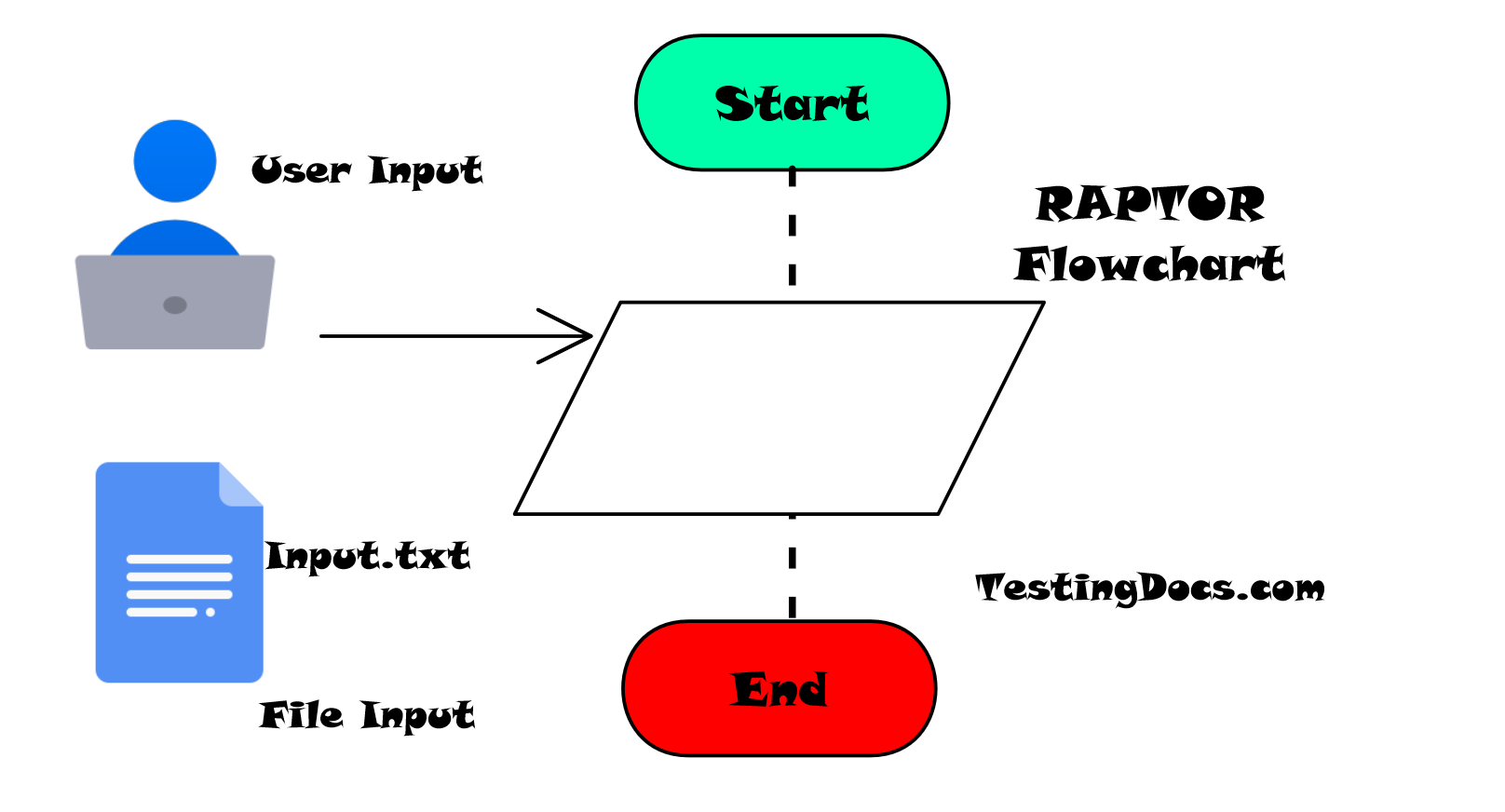# Read a File in the RAPTOR Flowchart

### Introduction

In this post, we will learn how to read a file in the Raptor flowchart. The standard Input flowchart symbol is used to read data from a file. The standard input to the flowchart is to prompt the user and the input is taken from the computer keyboard. We can override this behavior by Redirecting the input from the file.

### Redirect_Input

To read a file in the flowchart, we have to redirect the input to the file using
the function:

###After file processing we can turn off the redirection using the statement:

### Example

Let’s consider a sample input file that consists of the English alphabets each letter in a separate line. We will read the alphabets input file in the RAPTOR flowchart into an array and display them on to the console. At the last, we will display the count of alphabets present in the file.

A
B
C
D
E
F
G
H
I
J
K
L
M
N
O
P
Q
R
S
T
U
V
W
X
Y
Z

### PseudoCode

```   Public Sub Main()

Randomize

Dim raptor_prompt_variable_zzyz As String
Dim file
Dim count
Dim ARRAYSIZE
Dim line
ReDim names()

file = ""
ARRAYSIZE = 26
count = 1
line = ""
names(ARRAYSIZE) = ""
raptor_prompt_variable_zzyz = "Enter the filename"
file = InputBox(raptor_prompt_variable_zzyz)
redirect_input(file)
Do
raptor_prompt_variable_zzyz = ""
line = InputBox(raptor_prompt_variable_zzyz)
Loop Until line = ""
names(count) = line
MsgBox names(count)
count = count + 1
redirect_input(false)
MsgBox "Number of names:=" + (count - 1)

End Sub

```

### Writing to a file

https://www.testingdocs.com/writing-to-a-file-with-raptor/